Скачать презентацию Short Overview of Cryptography Lecture II John C

e0253ffed50b2b94c0b78525485da217.ppt

• Количество слайдов: 34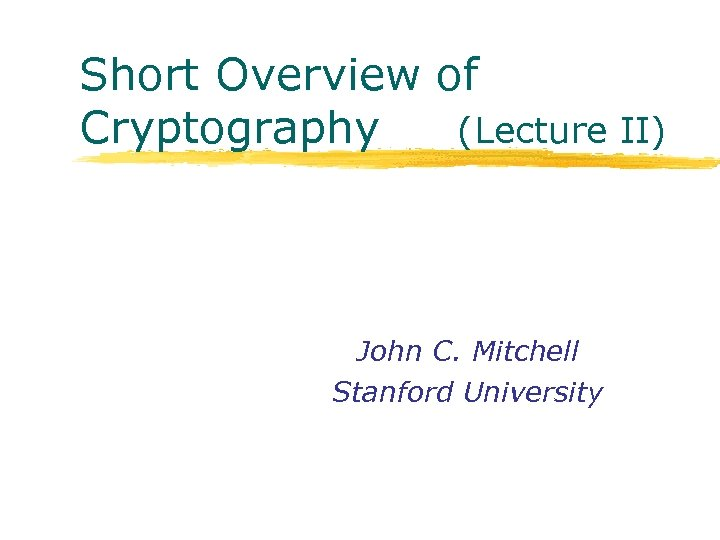Short Overview of Cryptography (Lecture II) John C. Mitchell Stanford UniversitySome philosophy l (my opinions) Do something useful with your life w Computers can do many things w Have fun! w Do something that matters l Learn something about the problems you solve w If you are going to do graphics, study visual art w If you work on computational biology, try to learn a little organic chemistry w If we are going to analyze security protocols, we should learn a few things about cryptography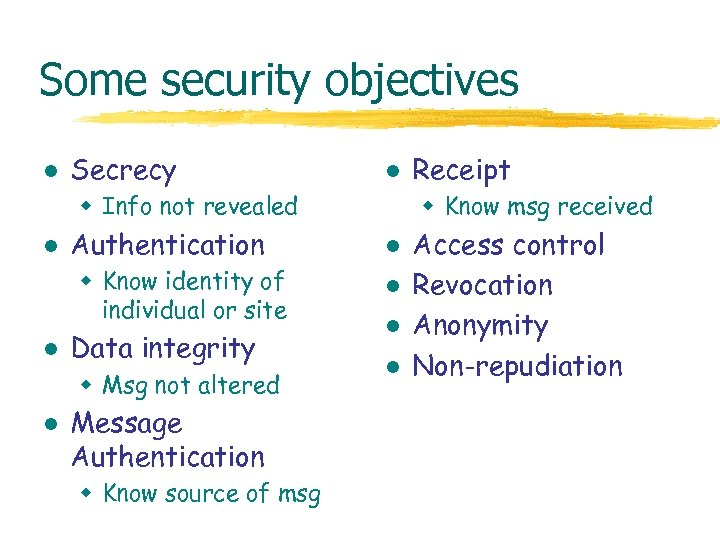Some security objectives l Secrecy l w Info not revealed l Authentication w Know identity of individual or site l Data integrity w Msg not altered l Message Authentication w Know source of msg Receipt w Know msg received l l Access control Revocation Anonymity Non-repudiation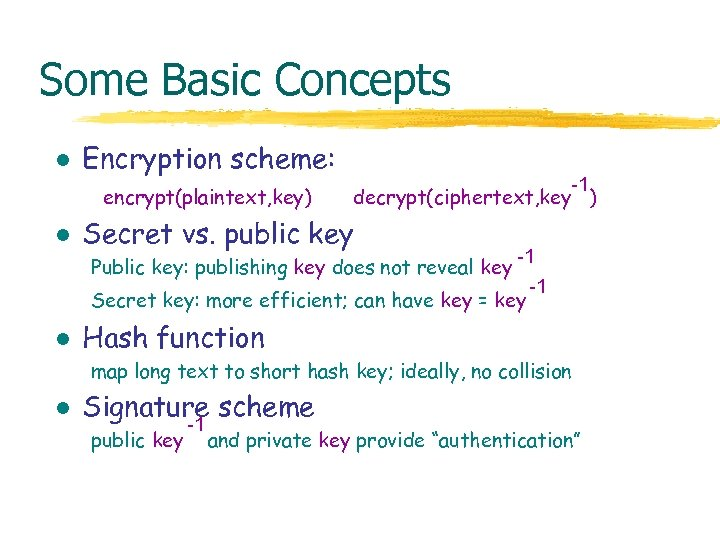Some Basic Concepts l Encryption scheme: encrypt(plaintext, key) l -1 decrypt(ciphertext, key ) Secret vs. public key -1 -1 Secret key: more efficient; can have key = key Public key: publishing key does not reveal key l Hash function map long text to short hash key; ideally, no collision l Signature scheme public key -1 and private key provide “authentication”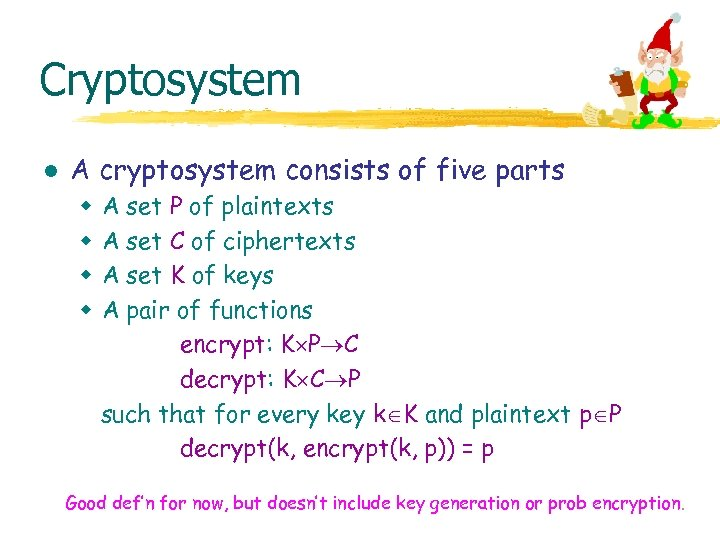Cryptosystem l A cryptosystem consists of five parts w w A set P of plaintexts A set C of ciphertexts A set K of keys A pair of functions encrypt: K P C decrypt: K C P such that for every key k K and plaintext p P decrypt(k, encrypt(k, p)) = p Good def’n for now, but doesn’t include key generation or prob encryption.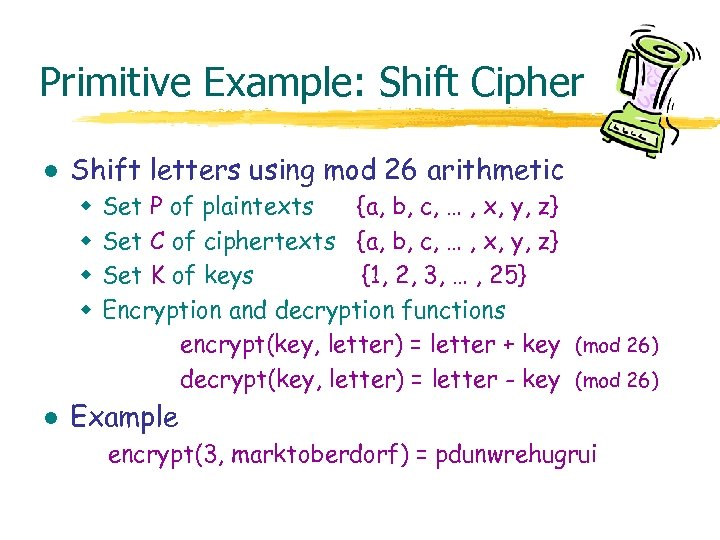Primitive Example: Shift Cipher l Shift letters using mod 26 arithmetic w w l Set P of plaintexts {a, b, c, … , x, y, z} Set C of ciphertexts {a, b, c, … , x, y, z} Set K of keys {1, 2, 3, … , 25} Encryption and decryption functions encrypt(key, letter) = letter + key (mod 26) decrypt(key, letter) = letter - key (mod 26) Example encrypt(3, marktoberdorf) = pdunwrehugrui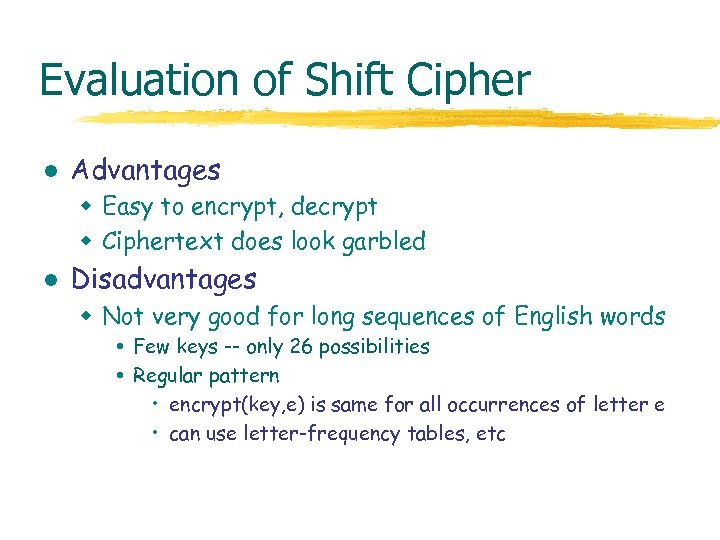Evaluation of Shift Cipher l Advantages w Easy to encrypt, decrypt w Ciphertext does look garbled l Disadvantages w Not very good for long sequences of English words Few keys -- only 26 possibilities Regular pattern • encrypt(key, e) is same for all occurrences of letter e • can use letter-frequency tables, etcLetter frequency in English l Five frequency groups [Beker and Piper] E has probability 0. 12 TAOINSHR have probability 0. 06 - 0. 09 DL have probability ~ 0. 04 CUMWFGYPB have probability 0. 015 - 0. 028 VKJXQZ have probability < 0. 01 Possible to break many letter-to-letter substitution ciphers.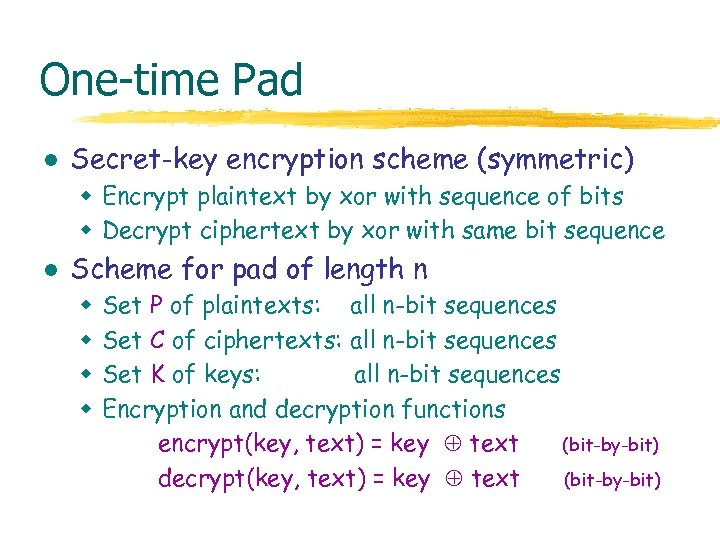One-time Pad l Secret-key encryption scheme (symmetric) w Encrypt plaintext by xor with sequence of bits w Decrypt ciphertext by xor with same bit sequence l Scheme for pad of length n w w Set P of plaintexts: all n-bit sequences Set C of ciphertexts: all n-bit sequences Set K of keys: all n-bit sequences Encryption and decryption functions encrypt(key, text) = key text (bit-by-bit) decrypt(key, text) = key text (bit-by-bit)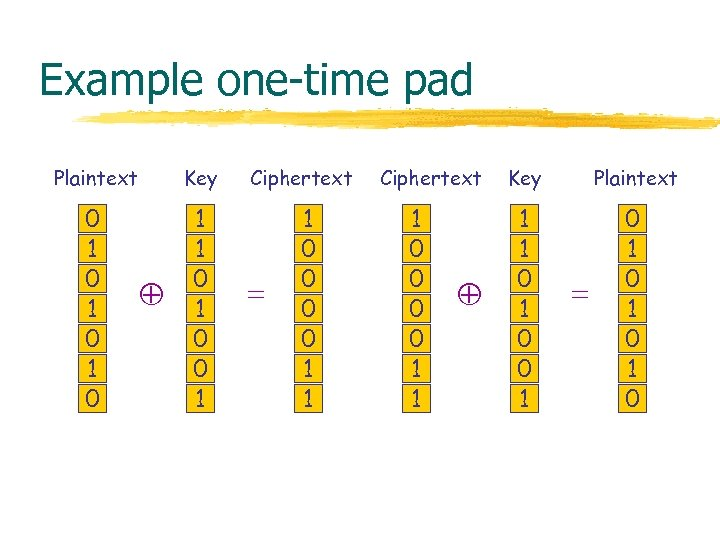Example one-time pad Plaintext Key 0 1 0 1 1 0 0 1 Ciphertext = 1 0 0 1 1 Ciphertext 1 0 0 1 1 Key Plaintext 1 1 0 0 1 0 1 0 =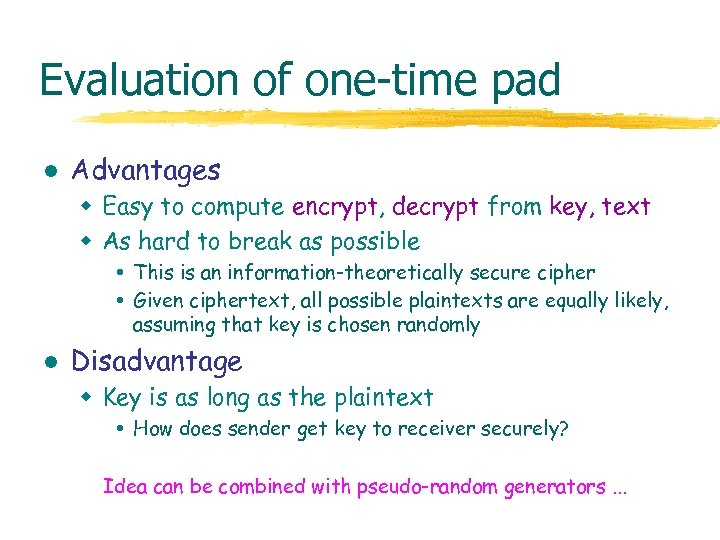Evaluation of one-time pad l Advantages w Easy to compute encrypt, decrypt from key, text w As hard to break as possible This is an information-theoretically secure cipher Given ciphertext, all possible plaintexts are equally likely, assuming that key is chosen randomly l Disadvantage w Key is as long as the plaintext How does sender get key to receiver securely? Idea can be combined with pseudo-random generators. . .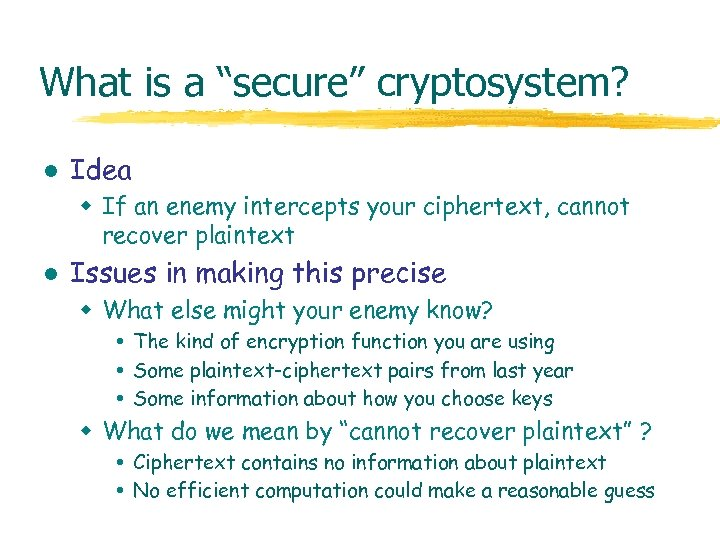What is a “secure” cryptosystem? l Idea w If an enemy intercepts your ciphertext, cannot recover plaintext l Issues in making this precise w What else might your enemy know? The kind of encryption function you are using Some plaintext-ciphertext pairs from last year Some information about how you choose keys w What do we mean by “cannot recover plaintext” ? Ciphertext contains no information about plaintext No efficient computation could make a reasonable guess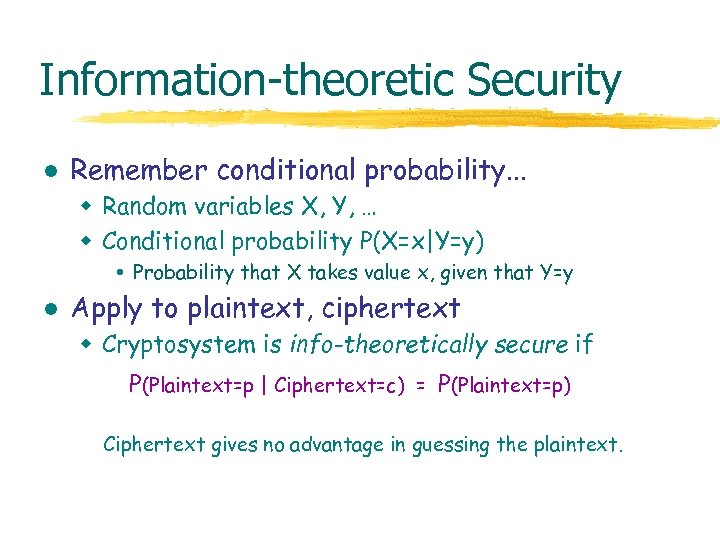Information-theoretic Security l Remember conditional probability. . . w Random variables X, Y, … w Conditional probability P(X=x|Y=y) Probability that X takes value x, given that Y=y l Apply to plaintext, ciphertext w Cryptosystem is info-theoretically secure if P(Plaintext=p | Ciphertext=c) = P(Plaintext=p) Ciphertext gives no advantage in guessing the plaintext.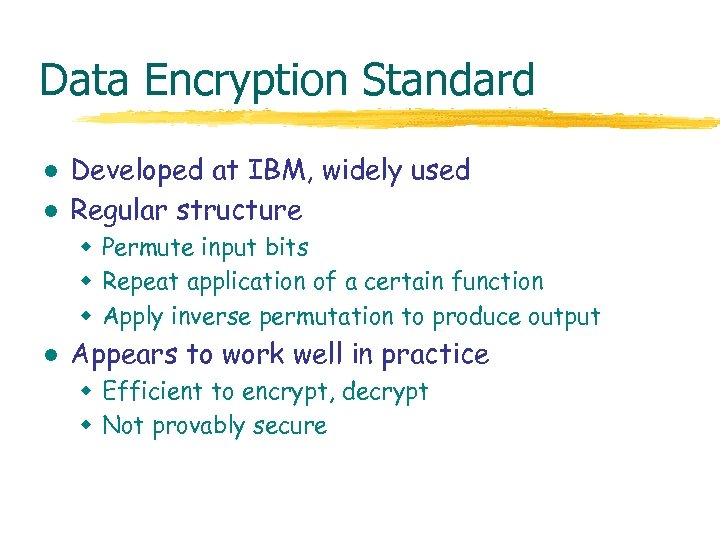Data Encryption Standard l l Developed at IBM, widely used Regular structure w Permute input bits w Repeat application of a certain function w Apply inverse permutation to produce output l Appears to work well in practice w Efficient to encrypt, decrypt w Not provably secure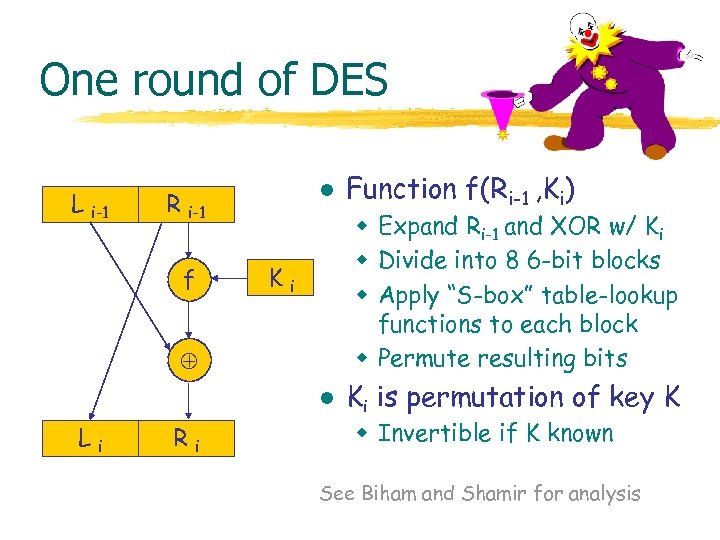One round of DES L i-1 l R i-1 f w Expand Ri-1 and XOR w/ Ki w Divide into 8 6 -bit blocks w Apply “S-box” table-lookup functions to each block w Permute resulting bits Ki l Li Ri Function f(Ri-1 , Ki) Ki is permutation of key K w Invertible if K known See Biham and Shamir for analysis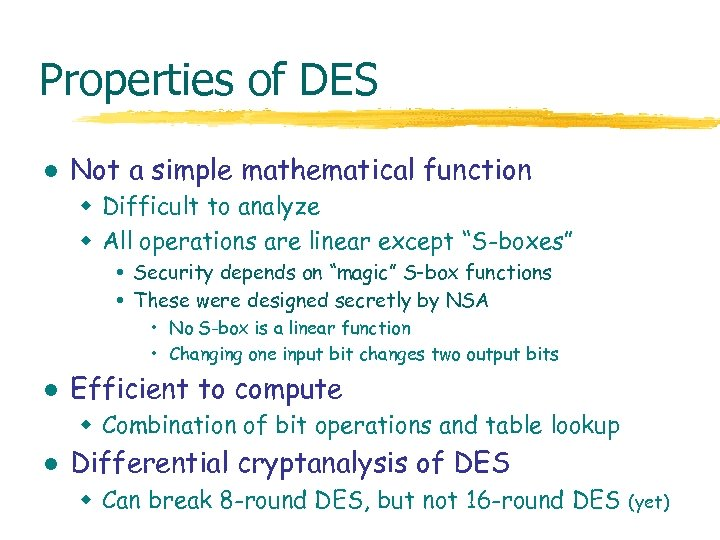Properties of DES l Not a simple mathematical function w Difficult to analyze w All operations are linear except “S-boxes” Security depends on “magic” S-box functions These were designed secretly by NSA • No S-box is a linear function • Changing one input bit changes two output bits l Efficient to compute w Combination of bit operations and table lookup l Differential cryptanalysis of DES w Can break 8 -round DES, but not 16 -round DES (yet)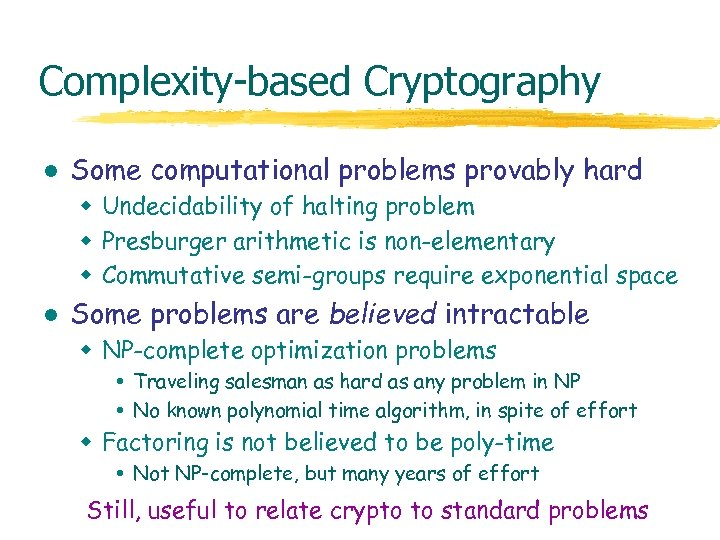Complexity-based Cryptography l Some computational problems provably hard w Undecidability of halting problem w Presburger arithmetic is non-elementary w Commutative semi-groups require exponential space l Some problems are believed intractable w NP-complete optimization problems Traveling salesman as hard as any problem in NP No known polynomial time algorithm, in spite of effort w Factoring is not believed to be poly-time Not NP-complete, but many years of effort Still, useful to relate crypto to standard problems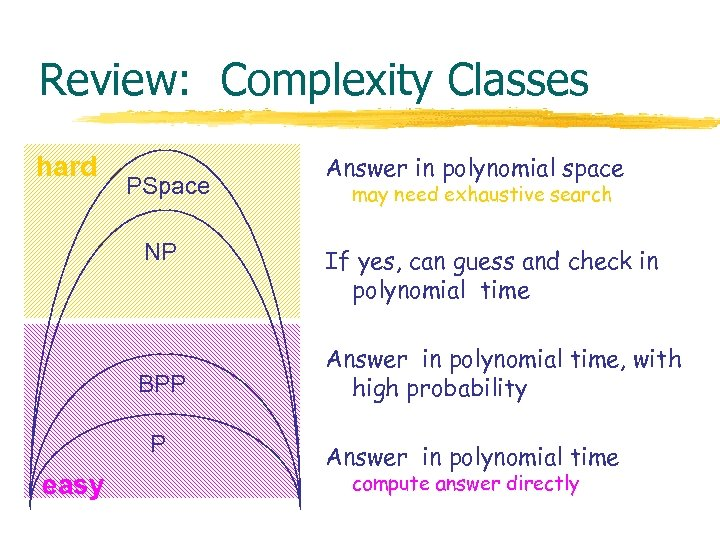Review: Complexity Classes hard PSpace NP BPP P easy Answer in polynomial space may need exhaustive search If yes, can guess and check in polynomial time Answer in polynomial time, with high probability Answer in polynomial time compute answer directly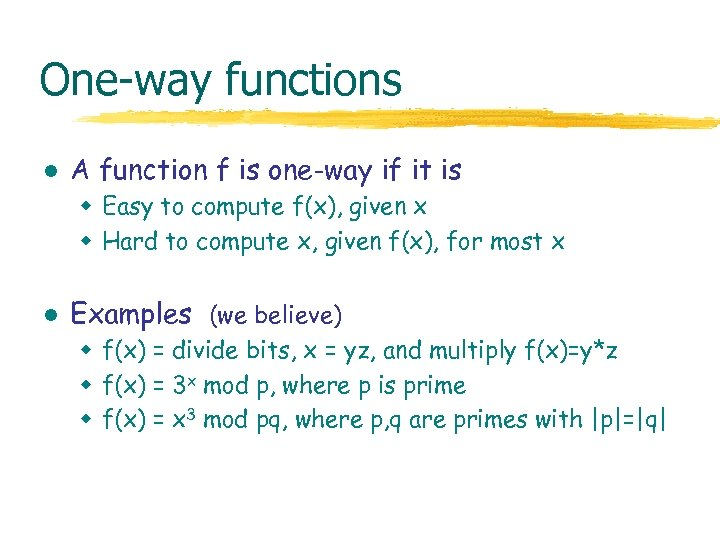One-way functions l A function f is one-way if it is w Easy to compute f(x), given x w Hard to compute x, given f(x), for most x l Examples (we believe) w f(x) = divide bits, x = yz, and multiply f(x)=y*z w f(x) = 3 x mod p, where p is prime w f(x) = x 3 mod pq, where p, q are primes with |p|=|q|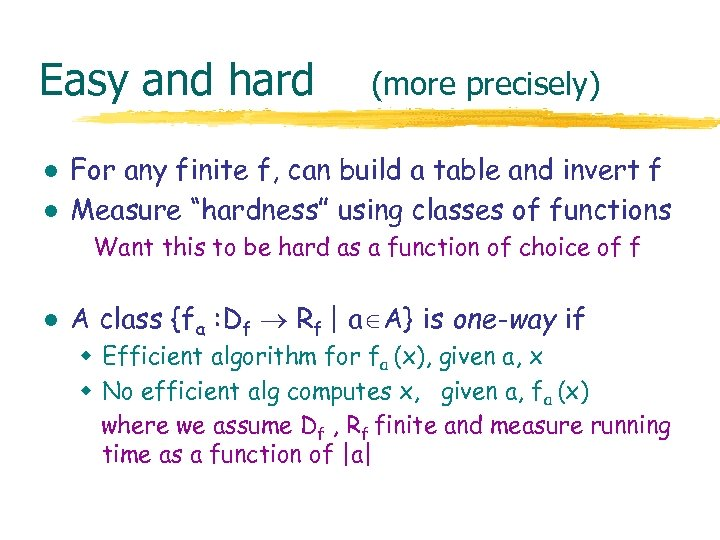Easy and hard l l (more precisely) For any finite f, can build a table and invert f Measure “hardness” using classes of functions Want this to be hard as a function of choice of f l A class {fa : Df Rf | a A} is one-way if w Efficient algorithm for fa (x), given a, x w No efficient alg computes x, given a, fa (x) where we assume Df , Rf finite and measure running time as a function of |a|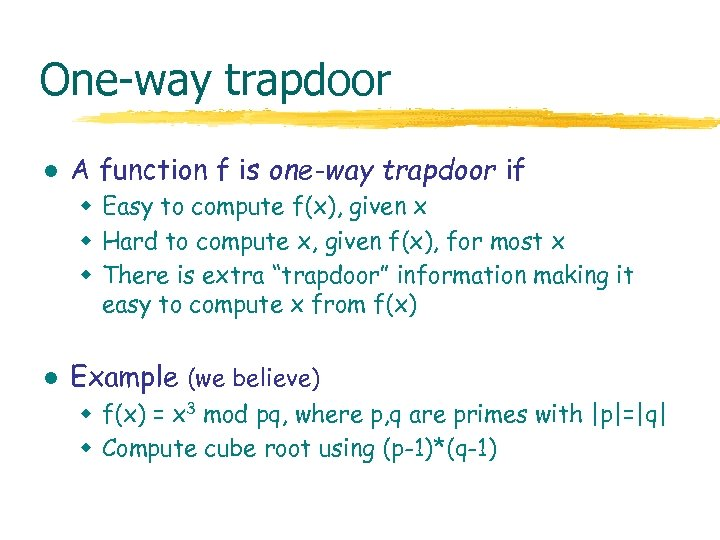One-way trapdoor l A function f is one-way trapdoor if w Easy to compute f(x), given x w Hard to compute x, given f(x), for most x w There is extra “trapdoor” information making it easy to compute x from f(x) l Example (we believe) w f(x) = x 3 mod pq, where p, q are primes with |p|=|q| w Compute cube root using (p-1)*(q-1)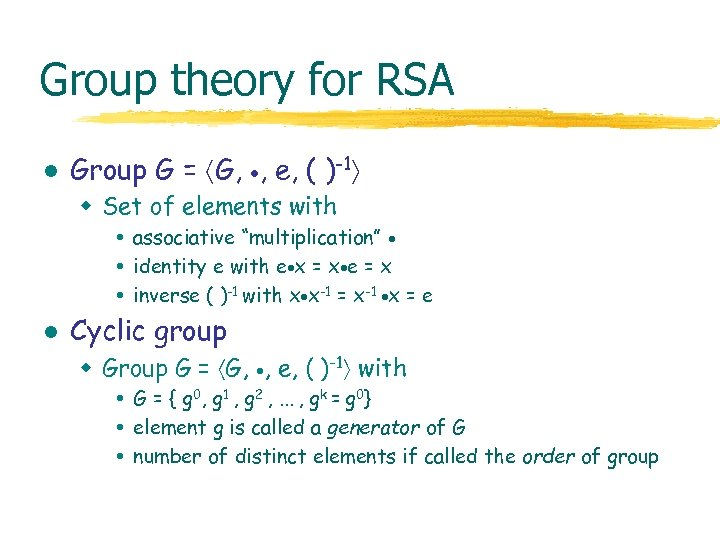Group theory for RSA l Group G = G, , e, ( )-1 w Set of elements with associative “multiplication” identity e with e x = x e = x inverse ( )-1 with x x-1 = x-1 x = e l Cyclic group w Group G = G, , e, ( )-1 with G = { g 0, g 1 , g 2 , . . . , gk = g 0} element g is called a generator of G number of distinct elements if called the order of group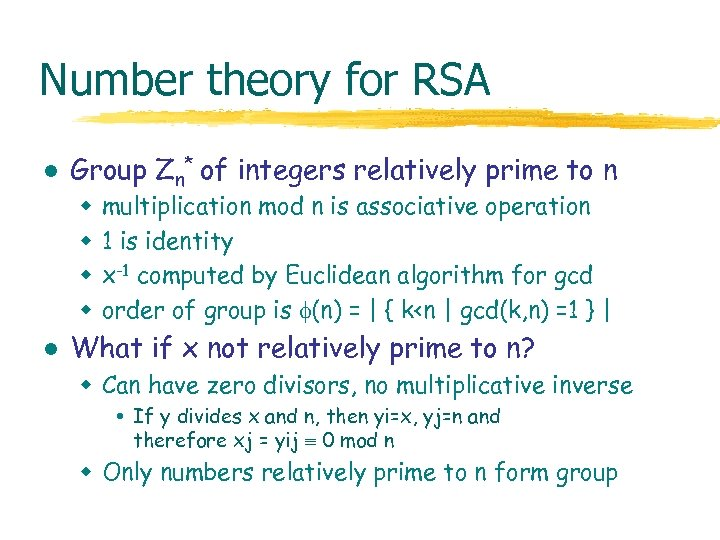Number theory for RSA l Group Zn* of integers relatively prime to n w w l multiplication mod n is associative operation 1 is identity x-1 computed by Euclidean algorithm for gcd order of group is (n) = | { k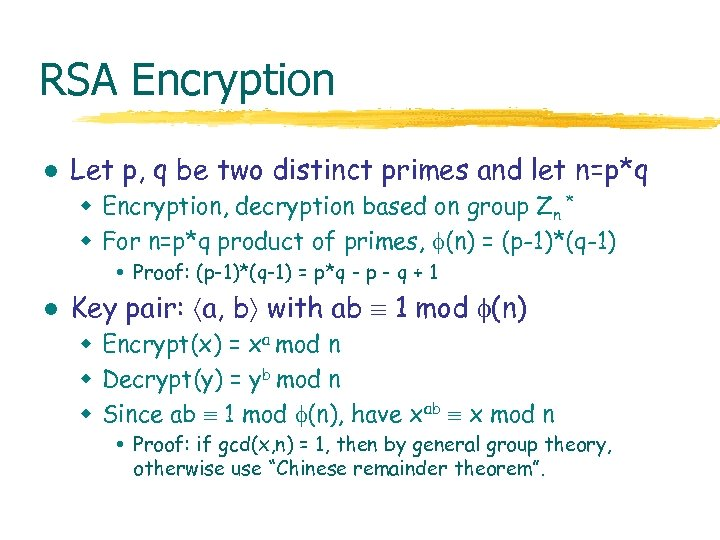RSA Encryption l Let p, q be two distinct primes and let n=p*q w Encryption, decryption based on group Zn * w For n=p*q product of primes, (n) = (p-1)*(q-1) Proof: (p-1)*(q-1) = p*q - p - q + 1 l Key pair: a, b with ab 1 mod (n) w Encrypt(x) = xa mod n w Decrypt(y) = yb mod n w Since ab 1 mod (n), have xab x mod n Proof: if gcd(x, n) = 1, then by general group theory, otherwise use “Chinese remainder theorem”.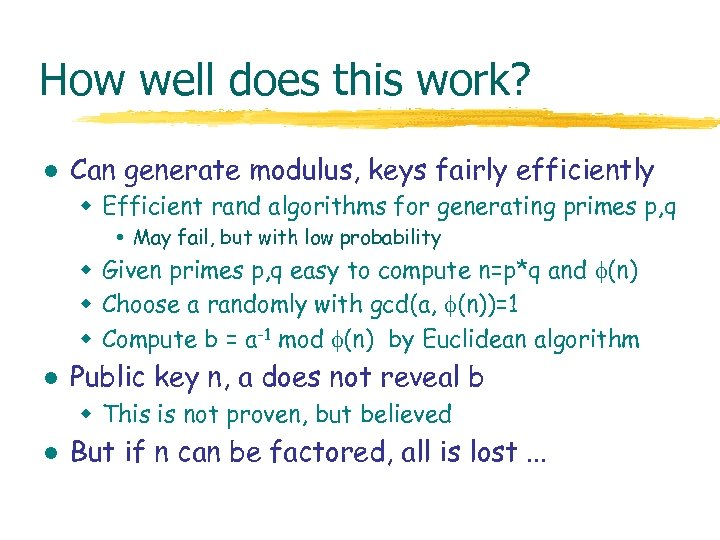How well does this work? l Can generate modulus, keys fairly efficiently w Efficient rand algorithms for generating primes p, q May fail, but with low probability w Given primes p, q easy to compute n=p*q and (n) w Choose a randomly with gcd(a, (n))=1 w Compute b = a-1 mod (n) by Euclidean algorithm l Public key n, a does not reveal b w This is not proven, but believed l But if n can be factored, all is lost. . .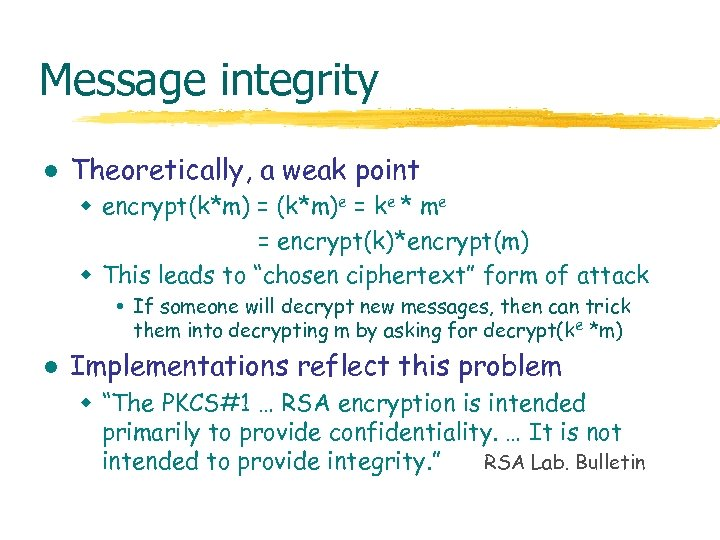Message integrity l Theoretically, a weak point w encrypt(k*m) = (k*m)e = ke * me = encrypt(k)*encrypt(m) w This leads to “chosen ciphertext” form of attack If someone will decrypt new messages, then can trick them into decrypting m by asking for decrypt(ke *m) l Implementations reflect this problem w “The PKCS#1 … RSA encryption is intended primarily to provide confidentiality. … It is not intended to provide integrity. ” RSA Lab. Bulletin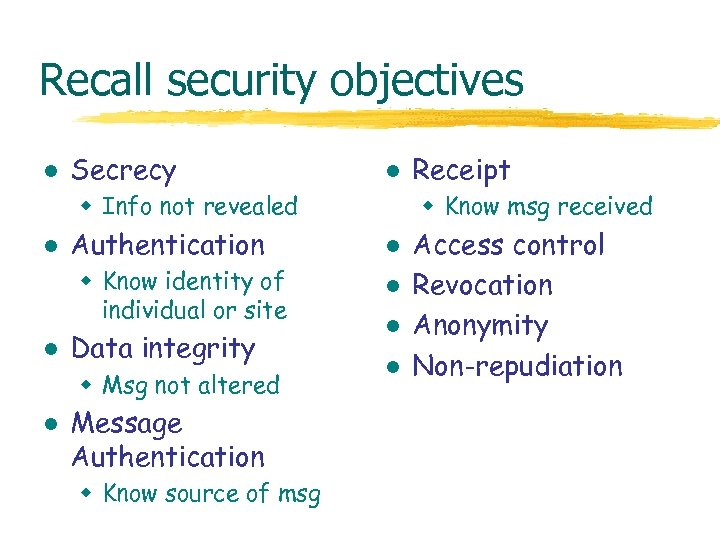Recall security objectives l Secrecy l w Info not revealed l Authentication w Know identity of individual or site l Data integrity w Msg not altered l Message Authentication w Know source of msg Receipt w Know msg received l l Access control Revocation Anonymity Non-repudiation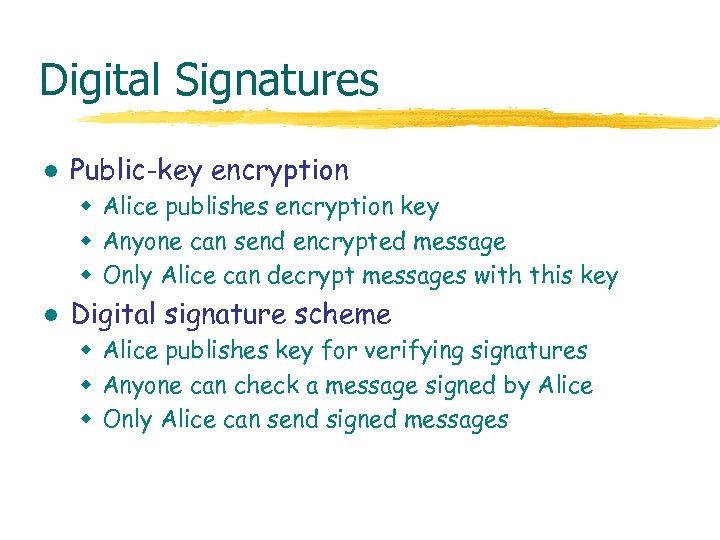Digital Signatures l Public-key encryption w Alice publishes encryption key w Anyone can send encrypted message w Only Alice can decrypt messages with this key l Digital signature scheme w Alice publishes key for verifying signatures w Anyone can check a message signed by Alice w Only Alice can send signed messages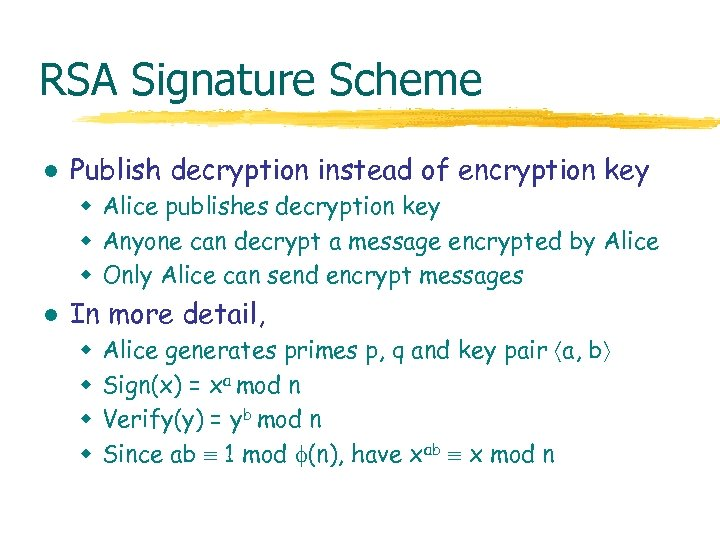RSA Signature Scheme l Publish decryption instead of encryption key w Alice publishes decryption key w Anyone can decrypt a message encrypted by Alice w Only Alice can send encrypt messages l In more detail, w w Alice generates primes p, q and key pair a, b Sign(x) = xa mod n Verify(y) = yb mod n Since ab 1 mod (n), have xab x mod n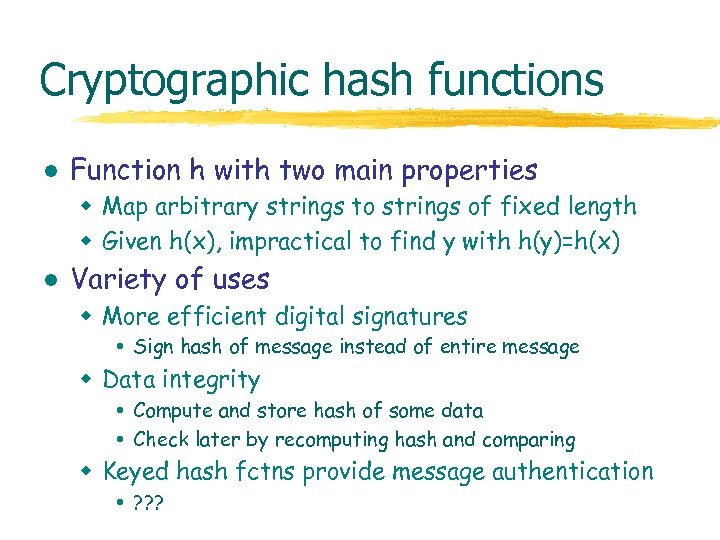Cryptographic hash functions l Function h with two main properties w Map arbitrary strings to strings of fixed length w Given h(x), impractical to find y with h(y)=h(x) l Variety of uses w More efficient digital signatures Sign hash of message instead of entire message w Data integrity Compute and store hash of some data Check later by recomputing hash and comparing w Keyed hash fctns provide message authentication ? ? ?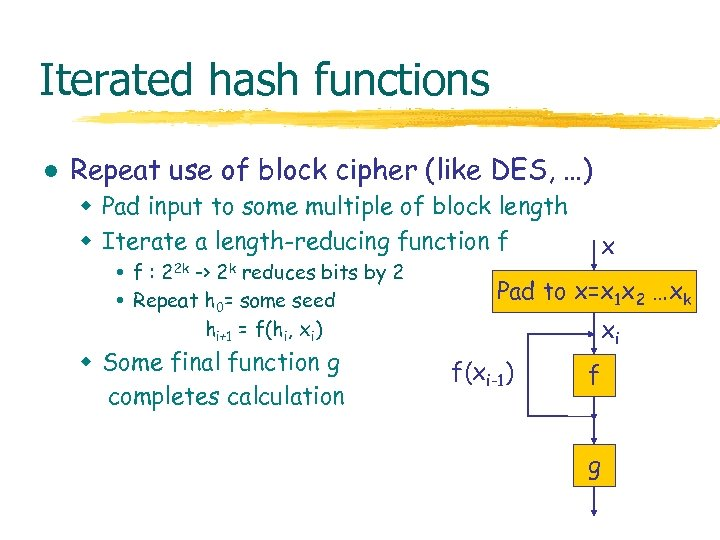Iterated hash functions l Repeat use of block cipher (like DES, …) w Pad input to some multiple of block length w Iterate a length-reducing function f f : 22 k -> 2 k reduces bits by 2 Repeat h 0= some seed hi+1 = f(hi, xi) w Some final function g completes calculation x Pad to x=x 1 x 2 …xk xi f(xi-1) f g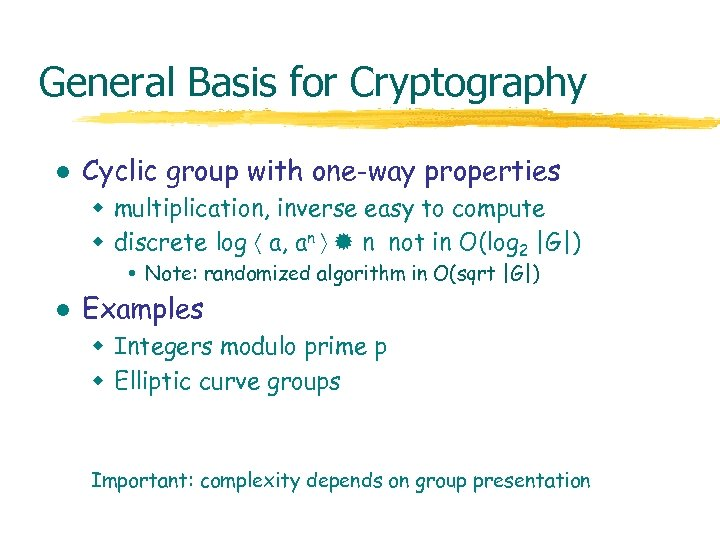General Basis for Cryptography l Cyclic group with one-way properties w multiplication, inverse easy to compute w discrete log a, an n not in O(log 2 |G|) Note: randomized algorithm in O(sqrt |G|) l Examples w Integers modulo prime p w Elliptic curve groups Important: complexity depends on group presentation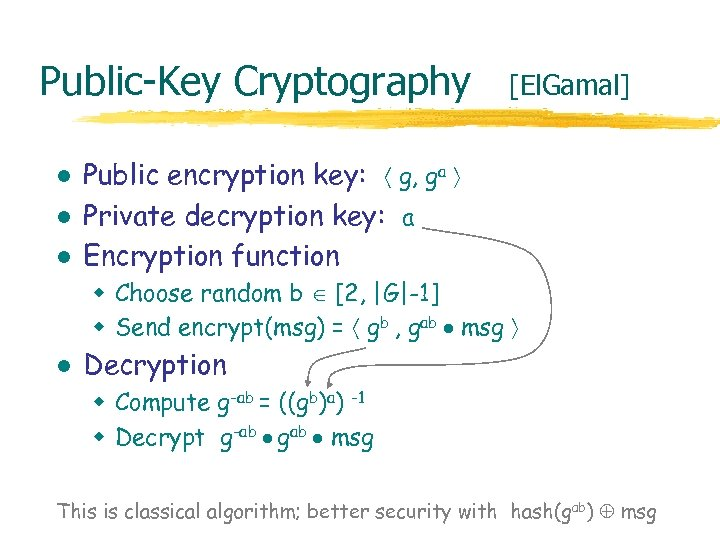Public-Key Cryptography l l l [El. Gamal] Public encryption key: g, ga Private decryption key: a Encryption function w Choose random b [2, |G|-1] w Send encrypt(msg) = gb , gab msg l Decryption w Compute g-ab = ((gb)a) -1 w Decrypt g-ab gab msg This is classical algorithm; better security with hash(gab) msg